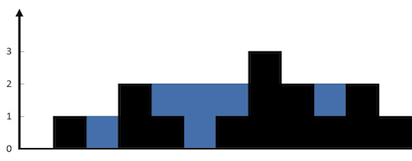# 凌云的博客

## LeetCode 算法题 42. Trapping Rain Water

### 题目

Given n non-negative integers representing an elevation map where the width of each bar is 1, compute how much water it is able to trap after raining.

For example,

``````Given [0,1,0,2,1,0,1,3,2,1,2,1], return 6.
``````### 解法

``````class Solution {
public:
int trap(vector<int>& height) {
int cap = 0;
int left = 0, right = height.size() - 1;
int maxLeft = 0, maxRight = 0;

while (left <= right) {
if (height[left] <= height[right]) {
if (height[left] >= maxLeft) {
maxLeft = height[left];
} else {
cap += (maxLeft - height[left]);
}

++left;
} else {
if (height[right] >= maxRight) {
maxRight = height[right];
} else {
cap += (maxRight - height[right]);
}

--right;
}
}

return cap;
}
};
``````